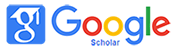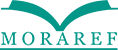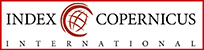### THE INFLUENCE OF MONOPOLY GAME TECHNIQUE ON MATHEMATICAL REASONING OF STUDENTS CLASS V OF MI / SD IN MATERIAL BUILD SPACE VOLUME

Khalimi Khalimi, Fery Muhamad Firdaus, Rihlah Fauziah

#### Abstract

This study aims to analyze the influence of monopoly game technique on mathematical reasoning of students of grade V of MI/SD in material build space volume. This research was conducted in MI Salafiyah Syafi'iyyah class V second semester of academic year 2017/2018 material volume of cube and beam building space. Monopoly game technique is a monopoly game that is modified as a tool for learning process with the addition of various questions in the game technique. The method used in this research is quasi experiment with research design of The Nonequivalent Pretest-Posttest Control Group, which involves a sample of 32 students of experimental class and 31 students of control class. Based on the posttest result of mathematical reasoning the students who were taught using monopoly game techniques were higher than the uneducated students using the monopoly game technique. This can be seen from the mean value of posttest of students' mathematical reasoning which is taught by monopoly game technique is equal to 77,72 and mean value of posttest of mathematical reasoning of student which not taught by monopoly game technique is equal to 70,32. From Mann Whitney U test results obtained results of 0.029 at significance level of 0.05 (0,000 ≤ 0,05). Then from the calculation of effect size, it is known that the effect size of this research is d = 0,572, so monopoly game technique has influence in medium category. Thus it can be concluded that the use of monopoly game techniques have an effect on mathematical reasoning of grade V students in MI Salafiyah Syafi'iyyah in the material volume of the cube and beam building space.

#### Keywords

Monopoly Game Technique; Learning Volume Build Cube Room and Beam; Student Mathematical Reasoning

#### Full Text:

PDF

DOI: http://dx.doi.org/10.32934/jmie.v2i2.74

### Refbacks

• There are currently no refbacks.